#### Bonding Theories and Molecular Geometry, Chemistry tutorial

Introduction

The VSEPR model is helpful for expecting molecular shape and estimating bond angles, but the model is insufficient for clarifying bond energy and bond strength.  Two other models, namely; the valence bond process and molecular orbital theory give a more precise and complex treatment of covalent bond formation that take into account bond energy and bond length. In the valence bond method, covalent bonds are assumed to be formed through overlaps of atomic orbitals. The kind of orbitals and the extent of the orbital overlap find out the bond length and bond energy. This model as well proposes the occurrence of hybridizations of atomic orbitals.

Valence bond theory

The valence bond theory states that 'a covalent bond is shaped via the overlap of 2 singly occupied atomic orbitals.' In Valence Bond Theory (VBT), bonding is versioned as occurring through the overlap of 2 atomic orbitals, one from each atom. Each atom's orbital encloses a single electron and a bond is shaped via the electrons, now paired in overlapping orbitals, holding the two nuclei mutually. Every covalent bond has a trait bond strength and bond length. The bond length is described as the distance between the 2 nuclei.

As an instance, the covalent bond in hydrogen fluoride is adequately described through the overlap of the 2 singly occupied atomic orbitals on each atom, as specified in Figure.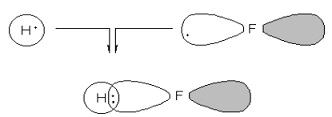Fig: The Covalent Bond in Hydrogen Fluoride is formed by the Overlap of the Singly Occupied Hydrogen 1s Orbital and the Singly Occupied Fluorine 2pz Orbital

Bond strength is described as the amount of energy required to homolytically break a bond (each of the nuclei obtains one of the two electrons from the bond).  A normal carbon-chlorine bond has a bond length of 1.78 Angstroms (1.78 X 10-10meters) and bond strength of (339 kJ/mol or 81 kcal/mol). Valence Bond Theory is helpful for understanding the basic idea following covalent bond formation. Though, it fails to illustrate the chemical bonding in easy organic molecules as methane (CH4). The electronic configuration of carbon, 1s22s22px22py12pz1, clearly indicates that carbon has only 2 singly occupied orbitals (2py and 2pz); therefore, carbon should only form two chemical bonds if this theory were rigorously correct. We know that carbon has a tendency to form 4 bonds, so something must be wrong through this theory.

In Molecular Orbital Theory (MOT), bonding is illustrated in expressions of the mathematical combination of atomic orbitals to form molecular orbitals. The recently formed orbitals are termed molecular orbitals since they belong to the complete molecule. The combination of 2 atomic orbitals leads to 2 molecular orbitals, a bonding molecular orbital and an antibonding molecular orbital.

Molecular orbital theory

Molecular Orbital Theory terms that:  a covalent bond is formed when the atomic orbitals of two interacting species merge to form an equivalent number of new molecular orbitals, so called since such new molecular orbitals encompass the complete molecule. Such molecular orbitals are formed via the mathematical addition/subtraction of various atomic orbitals.

As an example, the covalent bond in hydrogen gas is completely described by the combination of the two 1s atomic orbitals on each hydrogen atom. The new molecular orbitals (psi 1 and psi 2) are the consequence of the combination of the atomic orbitals in both an additive and subtractive sense as specified in Figure Psi 1 is termed a bonding molecular orbital, since electron density is centered between the 2 nuclei. Psi 2 is termed an anti-bonding orbital, since a node exists in the space between the two nuclei. As we will note from Figure 1, only the bonding molecular orbital is completely occupied, therefore forming the covalent bond.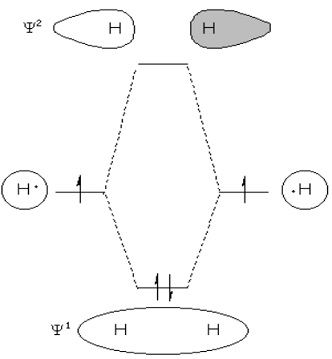Fig: The Covalent Bond in Hydrogen Gas is formed by the Combination of the Atomic Orbitals from Each Hydrogen Atom

Molecular Orbital Theory provides the most accurate picture of covalent chemical bonding to date. However, describing the molecular orbitals for even simple organic molecules like methane (CH4) precludes the use of this theory in any practical sense.

Hybridisation

The VBT and MOT can't effectively bond in organic molecules like methane (CH4). In order to describe these bonding situations, the idea of hybridisation has been introduced.

Hybridisation theory terms that:  a covalent bond is formed via the overlap of 2 singly occupied hybrid or atomic orbitals. Hybrid atomic orbitals are created by mixing together atomic orbitals to shape an equivalent number of new hybrid atomic orbitals. Hybrid orbitals are generally formed through mixing mutually 2s and 2p orbitals. Depending upon the number of 2p orbitals included, one can form sp, sp2, and sp3 hybrid orbitals.

A sp hybrid orbital is formed by mixing together the 2s and 2px atomic orbitals as shown in Figure. This will form 2 sp hybrid orbitals that are oriented with the major lobe of each pointing in opposite directions.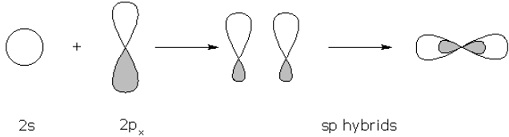Fig: Hybrid Orbitals formed by the Combination of 2s and 2px Atomic Orbitals

A sp2 hybrid orbital is formed through mixing mutually the 2s, 2px, and 2py atomic orbitals as illustration in Figure. This will form 3sp2 hybrid orbitals that are oriented through the major lobe of each pointing in a triangular fashion.Fig: Hybrid Orbitals formed by the Combination of 2s, 2px, and 2py Atomic Orbitals

A sp3 hybrid orbital is shaped via mixing together the 2s, 2px, 2py, and 2pz atomic orbitals as illustrate in Figure. This will form 4sp3 hybrid orbitals that are oriented through the major lobe of each pointing in a tetrahedral fashion.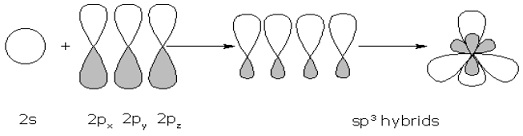Fig: Hybrid Orbitals formed by the Combination of 2s, 2px, 2py, and 2pz Atomic Orbitals

How are hybrid orbitals utilized? Well, consider methane (CH4). We know that carbon has 4 bonds, so we will use sp3 hybrid orbitals to overlap with the 1s orbitals of each hydrogen atom. Figure explains the procedure. Using this process, we can precisely explain the bonding in methane as being tetrahedral.Fig: Bonding in Methane using sp3 Hybrid Orbitals

We can as well utilize hybridisation theory to describe multiple bonds. Take ethene (C2H4) as an example. Each carbon atom has three atoms attached to it; therefore, we require 3 sp2 hybrid orbitals to account for the hydrogen attachments. The singly occupied, unhybridised 2pz orbital on each carbon will overlap, in a sideways manner, to provide the double bond. Figure 4.7 indicates this: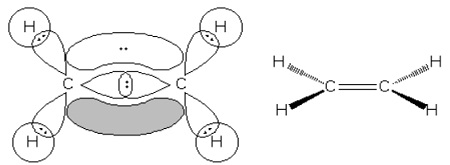Fig: Bonding in Ethene using sp2 Hybrid Orbitals

Hybridisation theory gives a helpful picture of covalent chemical bonding. It permits us to precisely expect the 3-dimensional shapes of molecules, as well as the accurate valency for each atom. Hybridisation theory as well provides us helpful information about the relative acidity of organic molecules depend upon the percentages character of a hybrid orbital.

Lewis structures

Lewis structures (as well recognized as Lewis dot diagrams, electron dot diagrams, and electron dot structures) are diagrams that show the bonding between atoms of a molecule and the lone pairs of electrons that might exist in the molecule. A Lewis structure can be drawn for any covalently bonded molecule, in addition to coordination compounds. The Lewis structure was termed after Gilbert Newton Lewis, who introduced it in his year 1916 article. The Atom and the Molecule. They are alike to electron dot diagrams in that the valence electrons in lone pairs are symbolized as dots, but they as well enclose lines to symbolize shared pairs in a chemical bond (single, double, triple, etc.).

Lewis structures demonstrate each atom and its position in the structure of the molecule using its chemical symbol. Lines are drawn between atoms that are linked to one another (pairs of dots can be utilized indeed of lines). Excess electrons, which form lone pairs are symbolized as pairs of dots, and are situated next to the atoms.

Even though many of the elements react via increasing, losing or sharing electrons until they have attained a valence shell electron configuration through a full octet (8) of electrons, there are many noteworthy exceptions to the "octet rule". Hydrogen (H) conforms instead to a duet rule wherein it fills its 1st (and outermost) shell by just 2 electrons or empties it entirely. Various compounds as boron trifluoride have unfinished orbitals while other these as sulphur hexafluoride, have a valence shell by more than 8 electrons.

Writing Lewis Structures by Trial and Error

The Lewis structure of a compound can be generated by trial and error. We start via writing symbols that contain the correct number of valence electrons for the atoms in the molecule. We then merge electrons to form covalent bonds until we come up by a Lewis structure in that all of the elements (with the exception of the hydrogen atoms) have an octet of valence electrons.

Instance: Let us apply the trial and error approach to generating the Lewis structure of carbon dioxide, CO2. We start via determining the number of valence electrons on each atom from the electron configurations of the elements. Carbon has 4 valence electrons, and oxygen has six.

C: [He] 2s2 2p2

O: [He] 2s2 2p4

We can symbolize this information as revealed at the top of the figure below. We now combine one electron from each atom to form covalent bonds between the atoms. When this is done, each oxygen atom has a total of 7 valence electrons and the carbon atom has a total of six valence electrons. Since none of these atoms have an octet of valence electrons, we merge another electron on each atom to form 2 more bonds. The consequence is a Lewis structure in that each atom has an octet of valence electrons.Fig: Lewis Structure of Carbon (IV) Oxide

A Step-by-Step Approach to Writing Lewis Structures

The trial-and-error process for writing Lewis structures can be time consuming. For all but the simplest molecules, the following step-by-step procedure is quicker.

Step 1:   Determine the total no of valence electrons

Step 2:   Write the skeleton structure of the molecule

Step 3:   Use 2 valence electrons to form each bond in the skeleton structure

Step 4:  Try to convince the octets of the atoms via distributing the continuing valence electrons as nonbonding electrons.

The 1st step in this procedure includes calculating the no of valence electrons in the molecule or ion. For a neutral molecule this is nothing more than the sum of the valence electrons on each atom. If the molecule carries an electric charge, we add one electron for each negative charge or subtract an electron for each positive charge.

Instance: Let us find out the no of valence electrons in the chlorate (ClO3-) ion.

A chlorine atom (Group VIIA) has seven valence electrons and each oxygen atom (Group VIA) has six valence electrons. Since the chlorate ion has a charge of -1, this ion encloses one more electron than a neutral ClO3 molecule. Therefore, the ClO3- ion has a total of 26 valence electrons.

ClO3-: 7 + 3(6) + 1 = 26

The 2nd step in this procedure involves deciding that atoms in the molecule are attached through covalent bonds. The formula of the compound often provides a hint as to the skeleton structure. The formula for the chlorate ion, for instance, proposes the given skeleton structure.The 3rd step supposes that the skeleton structure of the molecule is held mutually via covalent bonds. The valence electrons are hence separated into 2 categories: bonding electrons and nonbonding electrons.

Since it obtains 2 electrons to form a covalent bond, we can calculate the number of nonbonding electrons in the molecule by subtracting two electrons from the total number of valence electrons for each bond in the skeleton structure.  There are three covalent bonds in the most reasonable skeleton structure for the chlorate ion. As a consequence, six of the 26 valence electrons must be utilized as bonding electrons. This leaves 20 nonbonding electrons in the valence shell.

26 valence electrons

-   6 valence electrons

20 non-bonding electrons

The nonbonding valence electrons are now used to satisfy the octets of the atoms in the molecule. Each oxygen atom in the ClO3-ion previously has 2 electrons the electrons in the Cl-O covalent bond. Since each oxygen atom requires 6 nonbonding electrons to satisfy its octet, it takes 18 nonbonding electrons to satisfy the three oxygen atoms. This leaves one pair of nonbonding electrons that can be utilized to fill the octet of the central atom.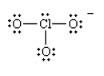Drawing skeleton structures

The hardest part of the 4-step procedure in the preceding section is writing the skeleton structure of the molecule. As a common rule, the less electronegative element is at the center of the molecule. Instance: The formulas of thionyl chloride (SOCl2) and sulphuryl chloride (SO2Cl2) can be translated into the subsequent skeleton structures.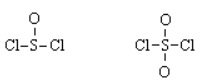It is as well helpful to recognize that the formulas for compound molecules are often written in a way that hints at the skeleton structure of the molecule.

Instance: Dimethyl ether is often written as CH3OCH3, which transforms into the subsequent skeleton structure: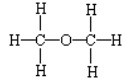Finally, it is helpful to recognize that many compounds that are acids obtain O-H bonds.

Instance: The formula of acetic acid is often written as CH3CO2H, since this molecule contains the following skeleton structure.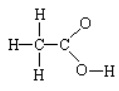Molecules that Contain Too Many or Too Few Electrons

Occasionally, we encounter a molecule that doesn't seem to have enough valence electrons. If we can't obtain a satisfactory Lewis structure through sharing a single pair of electrons, it might be possible to achieve this goal via sharing 2 or even 3 pairs of electrons.

Illustration: Consider formaldehyde (H2CO) that encloses 12 valence electrons.

H2CO: 2(1) + 4 + 6 = 12

The method of this molecule proposes the following skeleton structure.There are 3 covalent bonds in this skeletal structure that means that 6 valence electrons must be utilized as bonding electrons. This departs 6 nonbonding electrons. It is not possible, though, to convince the octets of the atoms in this molecule through only 6 nonbonding electrons. Whenever the nonbonding electrons are utilized to satisfy the octet of the oxygen atom, the carbon atom has a total of only 6 valence electrons.

We hence suppose that the carbon and oxygen atoms share 2 pairs of electrons. There are now 4 bonds in the skeleton structure that departs only four nonbonding electrons. This is sufficient, however, to satisfy the octets of the carbon and oxygen atoms. Every once in a while, we encounter a molecule for that it is impossible to write a satisfactory Lewis structure.

Illustration: Consider boron trifluoride (BF3) that contains 24 valence electrons.

BF3: 3 + 3(7) = 24

There are 3 covalent bonds in the most reasonable skeleton structure for the molecule. Since it takes six electrons to form the skeleton structure, there are 18 nonbonding valence electrons. Each fluorine atom requires six nonbonding electrons to satisfy its octet. Therefore, all of the nonbonding electrons are consumed through the 3 fluorine atoms. As a consequence, we run out of electrons while the boron atom has only 6 valence electrons.

The elements that form strong double or triple bonds are C, N, O, P, and S. Since neither boron nor fluorine drops in this category, we have to end by what appears to be an unsatisfactory Lewis structure.

Too many electrons

It is as well possible to encounter a molecule that seems to have too many valence electrons. When that happens, we expand the valence shell of the central atom.

Illustration: Consider the Lewis structure for sulphur tetrafluoride (SF4) which encloses 34 valence electrons.

SF4: 6 + 4(7) = 34

There are 4 covalent bonds in the skeleton structure for SF4. Since this needs using 8 valence electrons to form the covalent bonds that hold the molecule jointly, there are 26 nonbonding valence electrons.

Each fluorine atom requires 6 nonbonding electrons to convince its octet. Since there are four of these atoms, so we require 24 nonbonding electrons for this purpose. But there are 26 nonbonding electrons in this molecule. We have previously satisfied the octets for all 5 atoms, and we still have one more pair of valence electrons. We hence increase the valence shell of the sulphur atom to grip more than 8 electrons.

This increases an interesting question: How does the sulphur atom in SF4 hold 10 electrons in its valence shell? The electron configuration for a neutral sulphur atom seems to suggest that it takes 8 electrons to fill the 3s and 3p orbitals in the valence shell of this atom. But let us observe, once again, the selection rules for atomic orbitals.

According to such rules, the n = 3 shell of orbitals contains 3s, 3p, and 3d orbitals. Because the 3d orbitals on a neutral sulphur atom are all empty, one of such orbitals can be utilized to hold the extra pair of electrons on the sulphur atom in SF4

S: [Ne] 3s2 3p4 3d0

Resonance hybrids

For several molecules and ions, it is hard to find out that lone pairs should be shifted to form double or triple bonds. This is sometimes the case when multiple atoms of the similar kind enclose the central atom, and is particularly ordinary for polyatomic ions.

When this situation take places, the molecule's Lewis structure is said to be a resonance structure, and the molecule subsists as a resonance hybrid. Each of the different possibilities is superimposed on the others, and the molecule is considered to have a Lewis structure equivalent to an average of such states.

The nitrate ion (NO3-), for instance, must shape a double bond between nitrogen and one of the oxygen's to satisfy the octet rule for nitrogen. Though, since the molecule is symmetrical, it doesn't matter which of the oxygen's forms the double bond. In this case, there are 3 possible resonance structures. Expressing resonance when drawing Lewis structures might be done either via drawing each of the feasible resonance shapes and placing double-headed arrows between them or through using dashed lines to symbolize the partial bonds.

When comparing resonance structures for the similar molecule, generally those by the fewest formal charges contribute more to the overall resonance hybrid. When formal charges are required, resonance structures that have negative charges on the more electronegative elements and positive charges on the less electronegative elements are favored.

The resonance structure shouldn't be interpreted to point out the molecule switches between forms, but the molecule acts as the average of numerous forms.

The formula of the nitrite ion is NO2-.

Step 1: Nitrogen is the least electronegative atom, so it is the middle atom via numerous criteria.

Step 2: Count valence electrons. Nitrogen has 5 valence electrons; each oxygen has 6, for a total of (6 × 2) + 5 = 17. The ion has a charge of -1, which point out an extra electron, so the total no of electrons is 18.

Step 3: Place ion pairs. Each oxygen must be linked to the nitrogen that utilizes four electrons - 2 in each bond. The 14 remaining electrons should initially be placed as 7 lone pairs. Each oxygen might take a maximum of 3 lone pairs, giving each oxygen 8 electrons including the bonding pair. The 7th lone pair must be positioned on the nitrogen atom.

Step 4: Convince the octet rule. Both oxygen atoms now have 8 electrons allocated to them. The nitrogen atom has only 6 electrons assigned to it. One of the lone pairs on an oxygen atom must form a double bond, but either atom will work uniformly well. We hence must have a resonance structure.

Step 5: Bind up loose ends.  2 Lewis structures must be drawn: one through each oxygen atom double-bonded to the nitrogen atom. The 2nd oxygen atom in each structure will be single-bonded to the nitrogen atom. Situate brackets around each structure, and add the charge (-) to the upper right outside the brackets. Illustrate a double-headed arrow between the 2 resonance forms.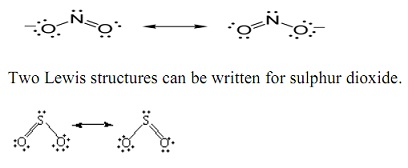The only variation between such Lewis structures is the identity of the oxygen atom to which the double bond is formed. As a consequence, they must be uniformly satisfactory symbolizations of the molecule.

Interestingly sufficient, neither of such structures is correct. The 2 Lewis structures propose that one of the sulphur-oxygen bonds is stronger than the other. There is no dissimilarity between the lengths of the two bonds in SO2, though that suggests that the two sulphur-oxygen bonds are equally strong.

When we can write more than one satisfactory Lewis structure, the molecule is an average, or resonance hybrid, of such structures. The meaning of the term resonance can be best understood through an analogy. In music, the reminders in a chord are often said to resonate they blend to provide something that is more than the sum of its parts. In a similar sense, the 2 Lewis structures for the SO2 molecule are in resonance. They blend to provide a hybrid that is more than the sum of its components. The fact that SO2 is a resonance hybrid of two Lewis structures is specified through writing a double-headed arrow between such Lewis structures, as subsequent in the figure above.

Formal charge

It is sometimes helpful to compute the formal charge on each atom in a Lewis structure. The 1st step in this computation includes dividing the electrons in each covalent bond between the atoms that form the bond. The no of valence electrons formally allocated to each atom is then evaluated through the number of valence electrons on a neutral atom of the element. If the atom has more valence electrons than a neutral atom, it is supposed to carry a formal negative charge. If it has fewer valence electrons, it is allocated a formal positive charge.

In terms of Lewis structures, formal charge is utilized in the explanation, comparison and assessment of likely topological and resonance structures  through determining the apparent electronic charge of each atom within depend upon its electron dot structure assuming exclusive covalence or non-polar bonding. It has utilizes in determining possible electron re-configuration when referring to reaction mechanisms, and often results in the same sign as the partial charge of the atom, through exceptions. In common, the formal charge of an atom can be computed using the subsequent formula, assuming non-standard descriptions for the mark-up utilized:

Cf = Nv - Ue - Bn

Where:

Cf   is the formal charge

Nv symbolizes the number of valence electrons in a free atom of the element

Ue signifies the number of unshared electrons on the atom

Bn characterizes the total number of bonds the atom has with another.

The formal charge of an atom is computed as the difference between the number of valence electrons that a neutral atom would have and the number of electrons that belong to it in the Lewis structure. Electrons in covalent bonds are split equally between the atoms involved in the bond.

The total of the formal charges on an ion should be equal to the charge on the ion, and the total of the formal charges on a neutral molecule should be the same to zero.

Shapes of Molecules

The valence shell electron pair repulsion theory

In order to predict the geometry of molecules, Nyholm and Gillespie increased a qualitative model recognized as Valence Shell Electron Pair Repulsion Theory (VSEPR Theory). The theory is a way of expecting the shape of a molecule based on the number of bonding and lone pairs of electrons in a polyatomic species.

It is depend on the fact that such electrons pairs interact and repel each other due the electrostatic repulsion. In doing so, they accept a spatial arrangement such that they are as far apart as possible and the electrostatic repulsion is minimized.

The basic suppositions of this theory are summarized below:

1.  The electron pairs in the valence shell around the central atom of a molecule repel each other and tend to orient in space so as to minimize the repulsions and maximize the distance between them.

2.  There are 2 kinds of valence shell electron pairs viz., (i) Bond pairs and (ii) Lone pairs.

Bond pairs are shared via 2 atoms and are attracted by two nuclei. Hence, they occupy less space and source less repulsion.

Lone pairs aren't included in bond formation and are in attraction through only one nucleus. Hence, they occupy more space.

As a consequence, the lone pairs cause more repulsion.

The order of repulsion between different types of electron pairs is as follows:

Lone pair - Lone pair > Lone Pair - Bond pair > Bond pair - Bond pair

As we know the bond pairs are generally symbolized through a solid line, while the lone pairs are symbolized via a lobe through 2 electrons.

3. In VSEPR theory, the numerous bonds are treated as if they were single bonds. The electron pairs in multiple bonds are treated together as a single super pair.

The repulsion caused via bonds raises through amplifies in the number of bonded pairs between    2 atoms for example a triple bond causes more repulsion than a double bond that in turn sources more repulsion than a single bond.

4. The form of a molecule can be expected from the number and kind of valence shell electron pairs around the central atom. When the valence shell of central atom encloses only bond pairs, the molecule supposes a symmetrical geometry due to even repulsions between them.

Though the symmetry is distorted when there are as well lone pairs along through bond pairs due to uneven repulsion forces.

5.  Primary and secondary effects on bond angle and shape:

(i) The bond angle reduces due to the presence of lone pairs that cause more repulsion on the bond pairs and as a consequence the bond pairs tend to come closer.

(ii) The repulsion between electron pairs rises through enhances in electronegativity of middle atom and therefore the bond angle enhances. The electronegative central atom attracts the bonding electrons toward itself, thereby shortening the distance between them. As a consequence, electron pair repulsion increases; hence the bonds tend to move away from each other. The bond pairs tend to shift away from each other since the distance between them is shortened as they are more localized on more electronegative middle atom.

Nevertheless the bond angle reduces whenever the electronegativities of peripheral atoms are more than that of central atom. There is enhancing in the distance between bond pairs since they are now closer to peripheral atoms.

As a consequence inter-electronic repulsions are decreased, and the bond angle is decreased.

The bond pairs tend to come closer since the distance between them is increased as they are more localized on more electronegative peripheral atoms.

(iii)   The bond angle decreases through increase in the size of central atom.

On smaller central atoms the bond pairs are closer and hence tend to move away from each other so as to minimize repulsion. Hence bond angle will be more.

On bigger central atoms, the bond pairs are more distant from each other and hence there is less repulsion. Hence they tend to move closer, thus decreasing the bond angle.

However the bond angle increases with increase in the size of peripheral atoms, which surround the central atom. There is less repulsion between smaller peripheral atoms and they can move closer to each other and thus decrease the bond angle.

There is a greater repulsion between bigger peripheral atoms and hence they tend to move away from each other. Thus bond angle increases.

Relation between Number and Type of Valence Electron Pairs with the Shape of Molecule

The shape of molecule and the approximate bond angles can be predicted from the number and kind of electron pairs in the valence shell of central atom as tabulated in table.

In table, the molecule is symbolized through 'AXE' notation, where

A = Central atom

X = Peripheral atom bonded to the central atom either by a single bond or by multiple bond;   indicating a bond pair.

E = Lone pair

As we know that

* The sum of number of peripheral atoms (X) and number of lone pairs (E) is also known as steric number.

Table: Relation between Number and Type of Valence Electron Pairs with the Shape of Molecule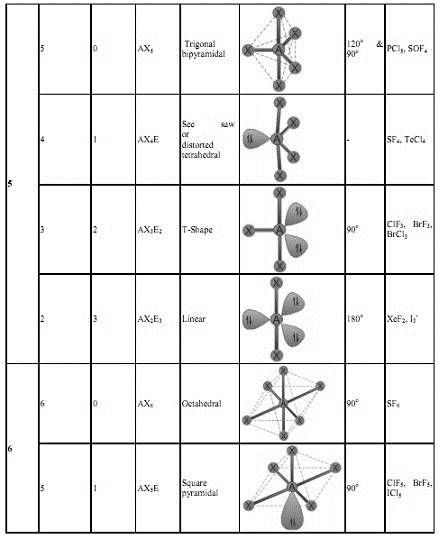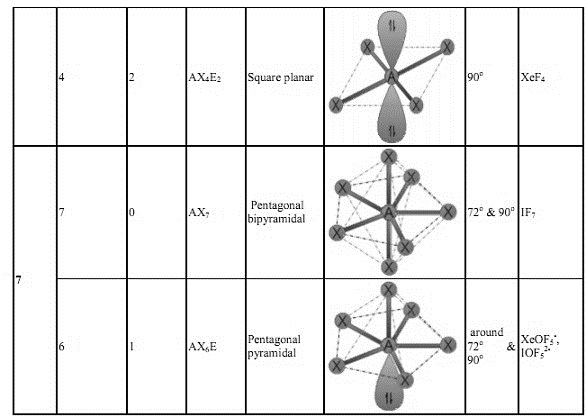Steps involved in predicting the Shapes of Molecules  using  VSEPR Theory

• The first step in determination of shape of a molecule is to write the Lewis dot structure of the molecule.
• Then find out the number of bond pairs and lone pairs in the valence shell of central atom. While counting the number of bond pairs, treat multiple bonds as if they were single bonds. Thus electron pairs in multiple bonds are to be treated collectively as a single super pair.
• Use the above table to predict the shape of molecule based on steric number and the number of bond pairs and lone pairs.

Applications and Illustrations of VSEPR theory methane (CH4)

The Lewis structure of methane molecule is: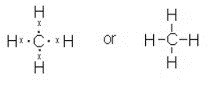• There are 4 bond pairs around the central carbon atom in its valence shell. Therefore it has tetrahedral shape by 109o 28' of bond angles.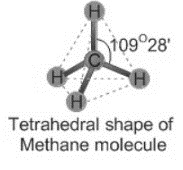Ammonia (NH3):

The Lewis structure of ammonia indicates there are 3 bond pairs and one lone pair around the middle nitrogen atom.• Since the steric number is 4, its structure is based on tetrahedral geometry. However, its shape is pyramidal with a lone pair on nitrogen atom.
• The bond angle is decreased from 109o28' to 107o48' due to repulsion caused via lone pair on the bond pairs.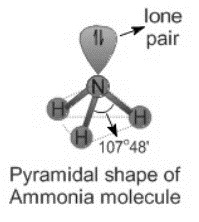Water (H2O)

It is evident from the Lewis structure of water molecule; there are two bond pairs and two lone pairs in the valence shell of oxygen. Hence its structure is based on tetrahedral geometry. However its shape is angular with two lone pairs on oxygen.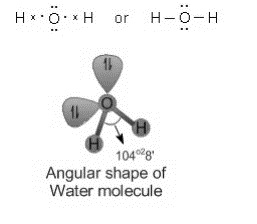The bond angle is reduced to 104o 28' due to repulsions caused through lone pairs on bond pairs. It can be noted that the bond angle reduces through enhance in the number of lone pairs on the central atom.

Sulphur tetrachloride (SCl4): Since there are 4 bond pairs and one lone pair around sulfur in its valence shell, the structure of SCl4 is based on trigonal bipyramidal geometry. It has seesaw shape with a lone pair occupying the equatorial position.

The angles between P-Claxial and P-Clequatorial are less than 90o due to repulsion exerted through the lone pair. The angle between P-Clequatorial bonds as well reduces from its usual value, 120o.

The lone pair occupies the equatorial position to minimize the repulsions.

As we know that usually the lone pairs, bulky groups and less electronegative atoms tend to occupy equatorial position to minimize repulsions. This is because they experience repulsion only from 2 groups at 90o, when they occupy the equatorial positions. However the repulsion will be more when they occupy axial positions, since they encounter three groups at 90o.  PF3Cl2:

There are only 5 bond pairs on phosphorus atom. Hence it has trigonal bipyramidal shape. The chlorine atoms occupy the equatorial positions to minimize the repulsions since they aren't only bulkier and as well less electronegative than fluorine atoms.

The bond pair of P-Cl is slightly closer towards the P atom when compared to the bond pair of P-F, since the chlorine atoms are moderately less electronegative than fluorine atoms. Therefore there is comparatively more negative charge accumulation towards P atom that creates the P-Cl bonds to experience more repulsion than P-F bonds. Therefore, they orient in equatorial positions at 120o to minimize repulsions.

As we know that, here we are comparing the polarity of P-Cl bond by P-F bond. But one should keep in brain that the bond pair of P-Cl bond is still closer to Cl, because it is more electronegative than P atom.

Formaldehyde (HCHO):

There are 3 bond pairs around the central carbon atom. The double bond between C and O is considered as a single super pair. Therefore, the shape of the molecule is trigonal planar and the bond angles are supposed to be equal to 120o

Nevertheless, the C=O exerts more repulsion on the C-H bond pairs. Therefore the ∠H-C-H bond angle will be less than 120o and the ∠H-C-O is greater than 120o.

Tutorsglobe: A way to secure high grade in your curriculum (Online Tutoring)